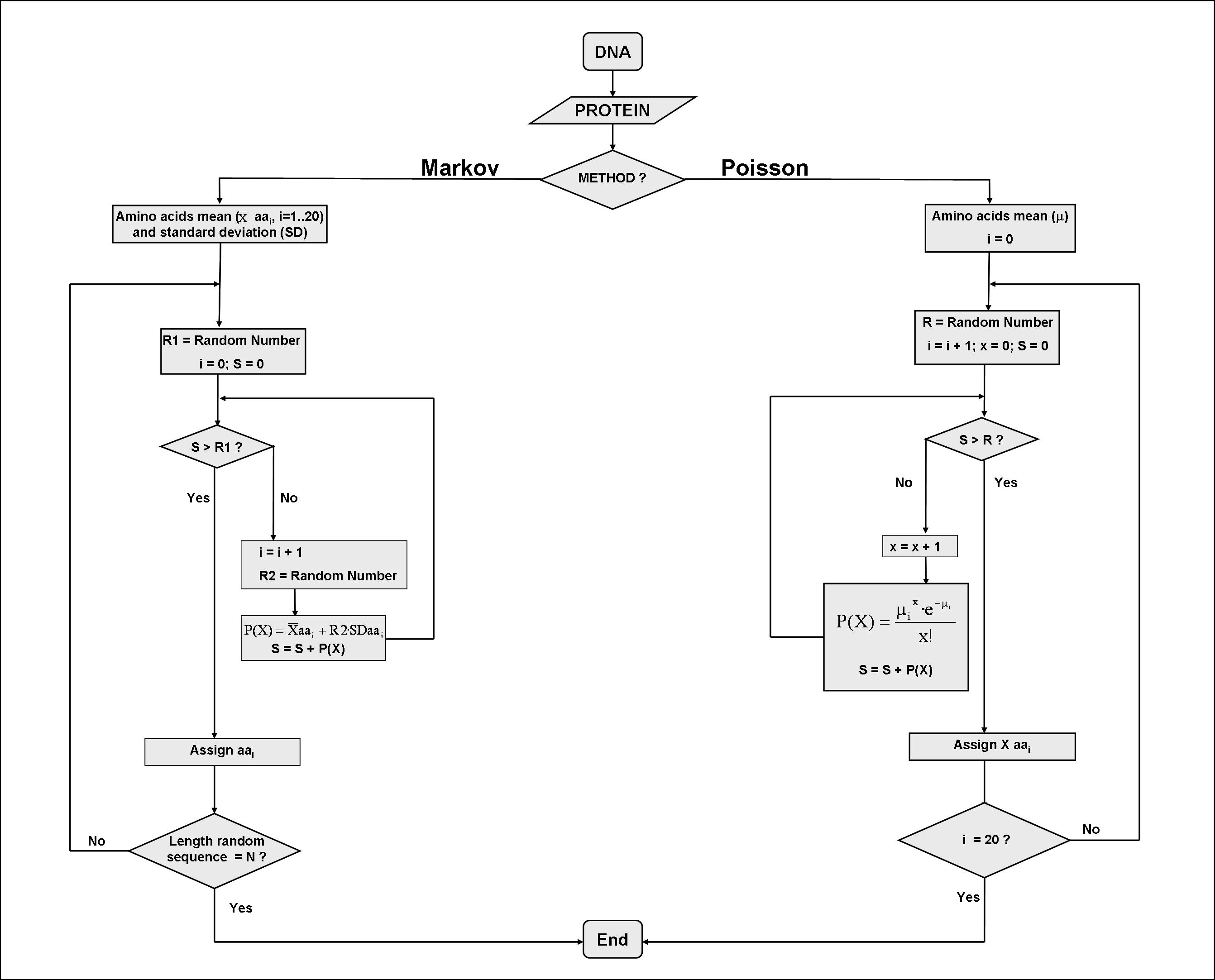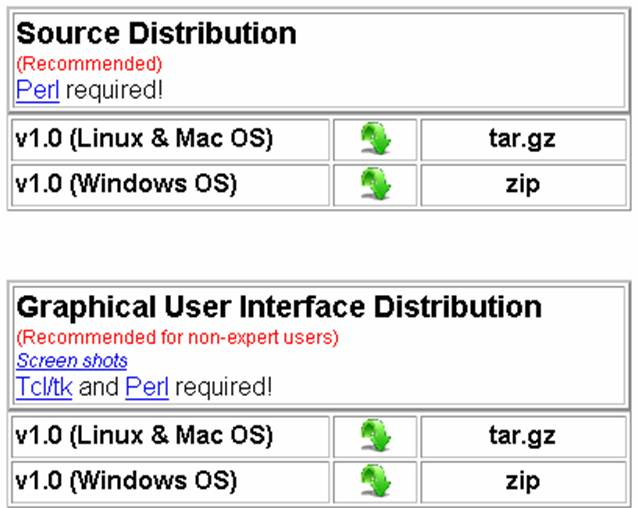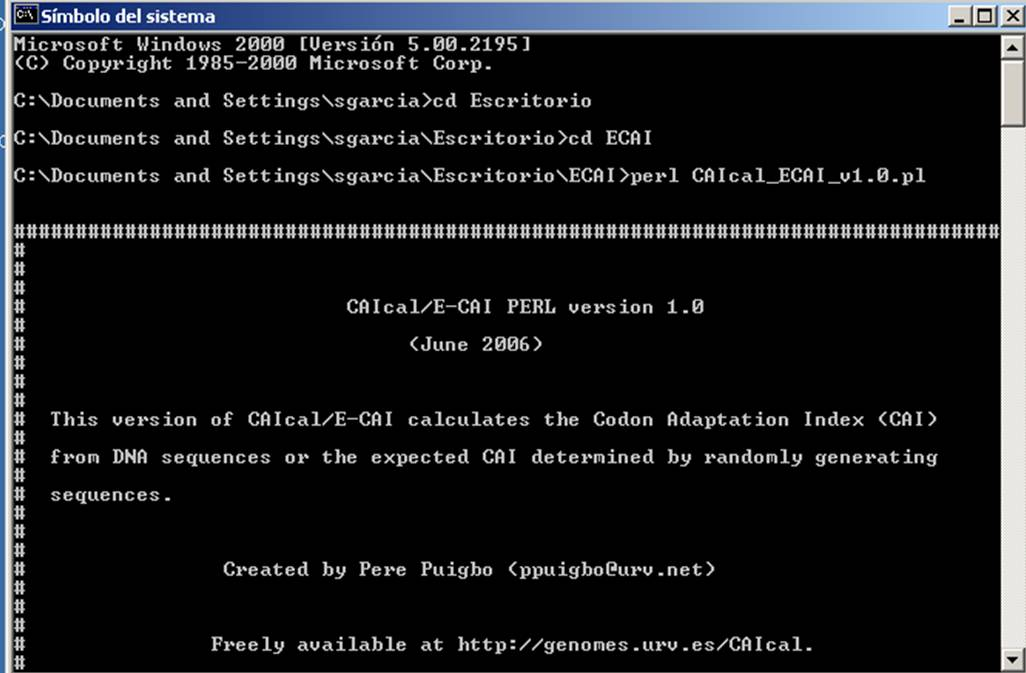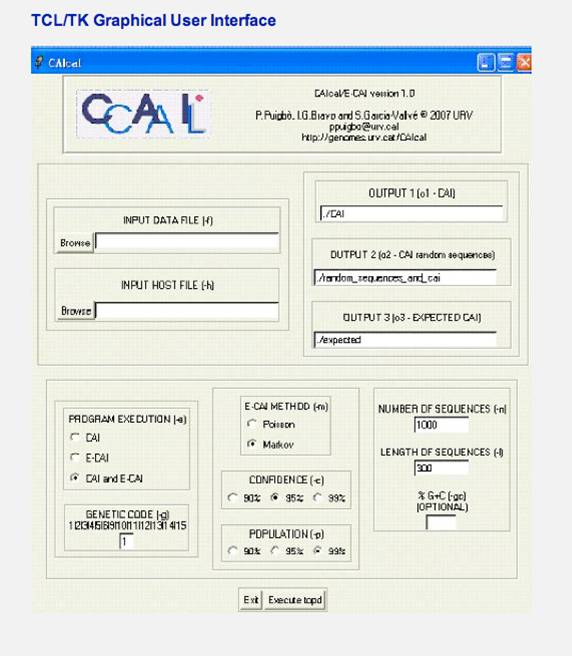Question 1: What are the requirements of the query sequence for calculating a CAI?

Question 2: Which codons are used to calculate the CAIs?

Question 3: Can I calculate a CAI for more than one sequence?

Question 4: Can I calculate a CAI without a codon usage reference table?

Question 5: Where can I find codon usage tables to use as a reference set?

Question 6: I have calculated the CAI of 3 sequences using human codon usage as a reference. The CAI of the sequences is 1) 0.535, 2) 0.856 and 3) 0.740. And the expected CAI for these sequences is 0.701 at 95% and 0.752 at 99%. How can I evaluate this result?

Question 7: I use Firefox 1.0.2 as navigator. Is there any problem to use CAIcal?

Question 8: How is the CAI along a sequence graphically represented?

Question 9: Can I graphically represent the CAI along a sequence using other programs?

Question 10: Why, in the graphical representation of the CAI along a sequence using a window size and a window step of one, are there some codons without a value or bar?

Question 11: I want to graphically represent the codon adaptation of a group of sequences that I have aligned. Do I need to use one or multiple reference sets?

Question 12: Is it important to maintain the same order of sequences between the protein alignment and the DNA sequences in the protein alignment section?

Question 13: Can I choose the window step and window length in the graphical representation of the CAI along a protein multialignment?

Question 14: How are the gaps considered in the graphical representation of the CAI along a protein multialignment?

Question 15: What are the differences between the Markov and Poisson methods for estimating the expected CAI value?

Both methods are similar in the sense that, using the amino acid composition of the query sequences and random generated numbers, they generate a series of amino acid sequences that are compositionally equivalent to the query. These sequences are then back-translated to DNA using the mean G+C content of the query. The CAI of these random-generated sequences are then used to estimate an expected value of CAI. Both methods provide similar results. The differences between the two methods can be seen in the following diagram:Question 16: What are the significance level and coverage in the estimation of an expected CAI

Question 17:What is the meaning of the Kolmogorov-Smirnov test in the estimation of the expected CAI?

Question 18: What is the meaning of the chi-square test in the results page of the estimation of an expected CAI?

Question 19: Is it possible to calculate the eCAI value one by one for many sequences instead of a set of sequences at one time?

The v1.1 of the source code and the graphical user interface distribution provide such output. Using the -s option, potential users can decide to calculate an unique eCAI value for a set of sequences (-s n) or to calculate, one by one, an eCAI value for each sequence(-s y).

Question 20: I suggest the authors to integrate the method used to analyze the difference between CAI and eCAI into their server.

Question 21: Is there a Windows version of the local version of E-CAI?After installing the Perl interpreter, downloading and uncompressing the source code file, windows users need to open a DOS-terminal, access to the directory that contain the source code and execute the script by writing "perl CAIcal_ECAI_v*.pl" (Replace * for the appropriate version).In addition, we have created a graphical interface in Tcl/Tk. Windows users only need to install the windows version of the Tcl/Tk toolkit (a link is provided in the download section), download the Tcl/Tk version of the E-CAI, unzipped and double click on the CAIcal.tcl file. The Tcl/Tk graphical interface of the E-CAI local version looks like this when run on Windows:Question 22: Which are the differences between the 1.0 and 1.1 local versions?

Question 23: Is there any difference between the CAI calculation through the web server and the local version?

Question 24: Have you ever compared the values of CAI calculated in CAIcal with other similar servers?

Yes, we have calculated the CAI of more than a thousand of DNA sequences using CAIcal and using CAIcalculator2 (Wu et al., 2005). Though, CAIcalculator2 uses the original algorithm proposed by Sharp and Li (1987) and CAIcal uses this algorithm with a few modifications proposed by Xia (2007), the results from both servers are comparable. Click here to see results from this test (sequences and reference set are also available to repeat the test).

Question 25: Where can I get a complete documentation about the source code of the local version?

Question 26: Which are the differences between the 1.1 and 1.2 local versions?

Question 27: Which are the differences between the 1.2 and 1.3 local versions?

Question 28: What are the differences between the Markov and Poisson methods for estimating the expected RCDI value?

Both methods are similar in the sense that, using the amino acid composition of the query sequences and random generated numbers, they generate a series of amino acid sequences that are compositionally equivalent to the query. These sequences are then back-translated to DNA using the mean G+C content of the query. The RCDI of these random-generated sequences are then used to estimate an expected value of RCDI. Both methods provide similar results. The differences between the two methods can be seen in the following diagram:Question 29: What are the significance level and coverage in the estimation of an expected RCDI

Question 30:What is the meaning of the Kolmogorov-Smirnov test in the estimation of the expected RCDI?

Question 31: What is the input table format?

An easy way to introduce the codon usage reference table in RCDI/eRCDI is to copy and paste the codon usage tables from Codon Usage Database (Nakamura et al., 2000). We have therefore added a link to this database (and also codon usage tables from model organisms) in the left frame of the server.

The codon usage table from the codon Usage Database format allowed in RCDI is as follows:

Fields: [triplet] [frequency: per thousand] ([number])...

We have also introduced another format as follows:

Question 32: What does the "Insert %G+C content" option mean?

Question 33:What are the results shown in the "gene's parameters table ?

The first and second columns contain the name of the gene and the RCDI respectivelly. Then, the frequency of each codon calculated as [(CiFa/CiFh)Ni] (where CiFa is the relative frequency of codon i for a specific amino acid in the test sequence; CiFh is the relative frequency of codon i for a specific amino acid in the reference sequence; Ni is the number of occurrences of codon i in the test sequence; and N, the total number of codons in the test sequence), and the last four columns contain the %G+C total and at each codon position.